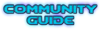## Question About Sector Lights

Ask about mapping, UDMF, using DoomBuilder/editor of choice, etc, here!

Moderator: GZDoom Developers

Forum rules
Before asking on how to use a ZDoom feature, read the ZDoom wiki first. If you still don't understand how to use a feature, then ask here.EnjayPosts: 26413
Joined: Tue Jul 15, 2003 4:58 pm
Location: Scotland

### Question About Sector Lights

When placing one if the dynamic sector light objects in a map, one of the parameters is "intensity scale". What does that actually do?

I mean, I know that the bigger the value, the wider the diameter of the light - but I can't figure out what the multiplication factor is.

For example, if I place a sector light in a sector that has light value 128, the dynamic light does not seem to have a radius of 128, if I give it an intensity scale of 1, or 256 if I give it a scale of 2, and so on; which is just as well, because those would generally be too big anyway. So I assume that the multiplication factor is less than 1. Even at that, the factor is a bit crude. I have often wanted to make something where factor 1 was a bit too small but 2 was a bit too big but the parameter can only be an int.

So, anyway, what is the maths behind the intensity scale parameter?
Moebius
Posts: 52
Joined: Thu Jan 28, 2021 1:45 am

### Re: Question About Sector Lights

I plugged some numbers in a spreadsheet and came up with this. Intensity = 4 is the default when you create a dynamic light. At Intensity = 4, light radius = Sector Brightness. At Intensity = 3 the Light radius is 3/4 the Sector Brightness. Intensity = 2 is 1/2. Intensity = 1 is 1/4. Getting bigger, Intensity = 5 light radius is 5/4 the Sector Brightness, etc..
Ex.
Sector Brightness = 168 with Dynamic Light Intensity at 6 would be 6/4*168 = 252 Light Radius.EnjayPosts: 26413
Joined: Tue Jul 15, 2003 4:58 pm
Location: Scotland

### Re: Question About Sector Lights

OK, I can follow that. Much appreciated, thanks.